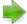#Pillow-Problems: Problem #68

Math Lair Home > Source Material > Pillow-Problems > Problem #68Problem #52 | Problem #70For more information on this collection, see Pillow-Problems by Charles L. Dodgson (Lewis Carroll).

Note: For information on pre-decimal British currency and coins, see pre-decimal British currency.

## Problem:

68.

Five friends agreed to form themselves into a Wine-Company (Limited). They contributed equal amounts of wine, which had been bought at the same price. They then elected one of themselves to act as Treasurer; and another of them undertook to act as Salesman, and to sell the wine at 10% over cost-price.

The first day the Salesman drank one bottle, sold some, and handed over the receipts to the Treasurer.

The second day he drank none, but pocked the profits on one bottle sold, and handed over the rest of the receipts to the Treasurer.

That night the Treasurer visited the Cellars, and counted the remaining wine. “It will fetch just £11,” he muttered to himself as he left the Cellars.

The third day the Salesman drank one bottle, pocked the profits on another, and handed over the rest of the receipts to the Treasurer.

The wine was now all gone: the Company held a Meeting, and found to their chagrin that their profits (i.e. the Treasurer's receipts, less the original value of the wine) only cleared 6d a bottle on the whole stock. These profits had accrued in 3 equal sums on the 3 days (i.e. the Treasurer's receipts for the day, less the original value of the wine taken out during the day, had come to the same amount every time); but of course only the Salesman knew this.

(1) How much wine had they bought? (2) At what price?

[28/2/89

68.

(1) 5 dozen; (2) 8/4 a bottle.

## Solution:

68.

Let the Nos of bottles, taken out on the 3 days, be ‘x, y, z’. Let each bottle have cost 10v pence, and therefore be sold for 11v pence.

Then the Treasurer's receipts, on the 3 days, were (x − 1) · 11v, y · 11vv, (z − 1) · 11vv; yielding, as profits (i.e. as remainders after deducting cost-price of bottles taken out), xv − 11v, yvv, zv − 12v. Then these 3 quantities are equal.

Hence y = x − 10, and z = x + 1;

∴ total No. of bottles, being (x + y + z), = 3x − 9.

Now total profits are (x + y + z) · v − 24v; i.e. (3x − 33)v;

∴ profit, per bottle =
 ((3x − 33) · v)/ (3x − 9)
; and this must = 6;

∴ (x − 11) · v = (x − 3) · 6.

Also, z · 11v = 11 × 240; i.e. (x + 1) · 11v = 11 × 240;

 (x − 11)/ (x + 1)
=
 (6 · (x − 3))/ (240)
;

∴ (x + 1) · (x − 3) = 40 · (x − 11);

x² − 2x − 3 = 40x − 440;

x² − 42x + 437 = 0.

Now 42² − 4 × 437 = 1764 − 1748 = 16;

x =
 (42 ± 4)/ (2)
= 24 or 19;

∴ No. of bottles = 60 or 48; but it is a multiple of 5; ∴ it = 60.

Also, (x + 1) · 11v = 11 × 240; i.e. 24v = 240;

v = 10;

i.e. the wine was bought @ 8/4 a bottle, and sold @ 9/2 a bottle.

Q.E.F.